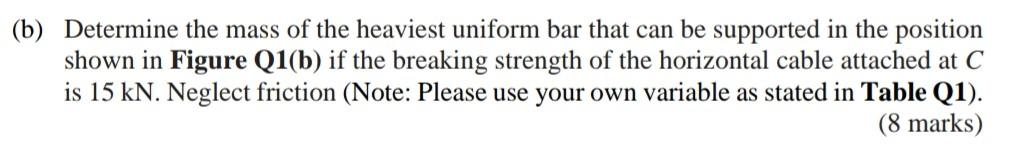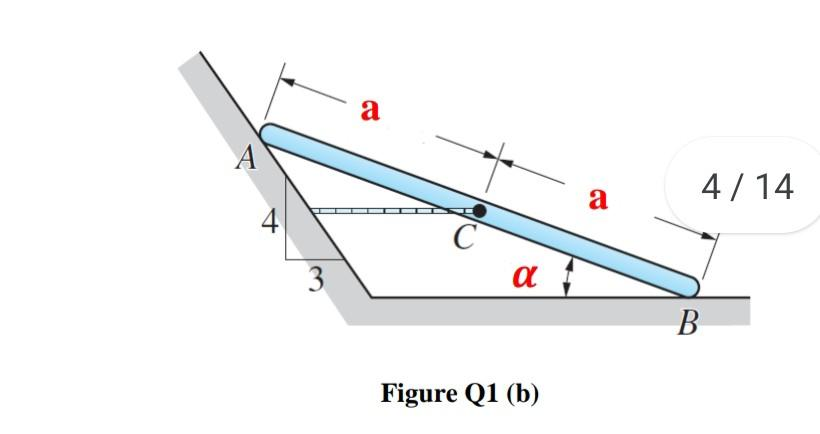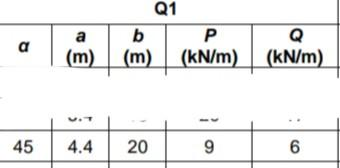# Question (b) Determine the mass of the heaviest uniform bar that can be supported in the position shown in Figure Q1(b) if the breaking strength of the horizontal cable attached at C is 15 kN. Neglect friction (Note: Please use your own variable as stated in Table Q1). (8 marks) а A 4/ 14 a С α 3 B Figure Q1 (b) Q1 b P Q (m) (m) (kN/m) (kN/m) a a a a 45 4.4 20 9 6SUAHCI The Asker · Civil EngineeringTranscribed Image Text: (b) Determine the mass of the heaviest uniform bar that can be supported in the position shown in Figure Q1(b) if the breaking strength of the horizontal cable attached at C is 15 kN. Neglect friction (Note: Please use your own variable as stated in Table Q1). (8 marks) а A 4/ 14 a С α 3 B Figure Q1 (b) Q1 b P Q (m) (m) (kN/m) (kN/m) a a a a 45 4.4 20 9 6
More
Transcribed Image Text: (b) Determine the mass of the heaviest uniform bar that can be supported in the position shown in Figure Q1(b) if the breaking strength of the horizontal cable attached at C is 15 kN. Neglect friction (Note: Please use your own variable as stated in Table Q1). (8 marks) а A 4/ 14 a С α 3 B Figure Q1 (b) Q1 b P Q (m) (m) (kN/m) (kN/m) a a a a 45 4.4 20 9 6